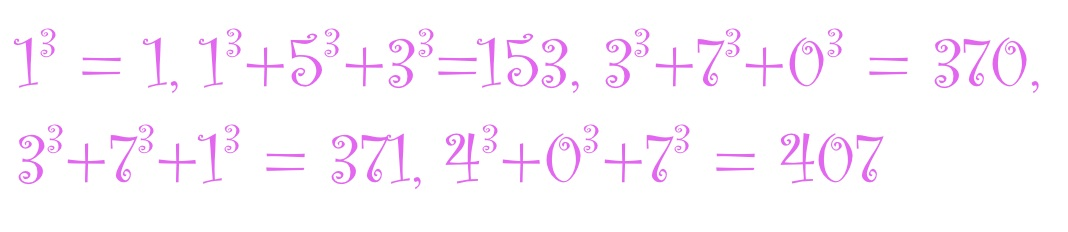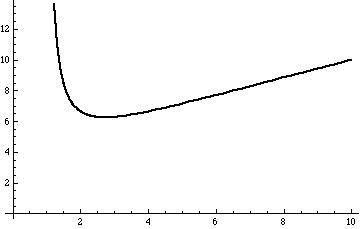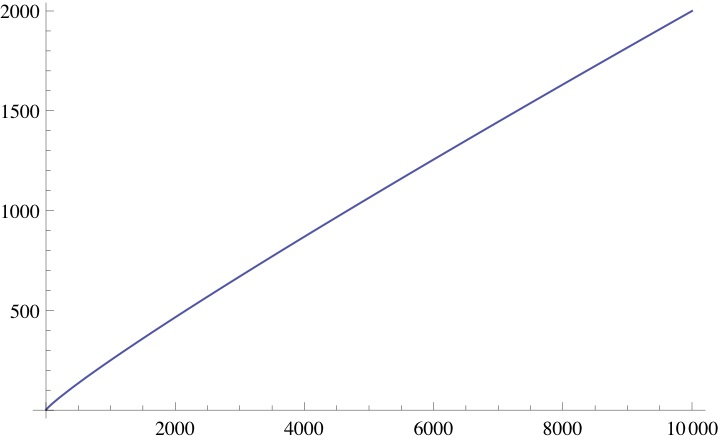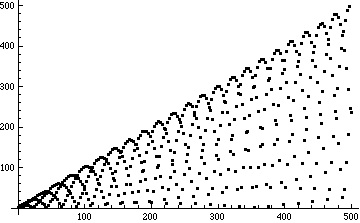# Factorions, Munchausen numbers, cubions and related things

Posted by: Gary Ernest Davis on: April 28, 2011

####Factorions

The four numbers \$latex 1, decease 2, treatment 145 extrm{ and } 40585\$ have the following peculiar property:

\$latex 1!=1, clinic 2!=2, 1!+4!+5!=145 extrm{ and } 4!+0!+5!+8!+5! =40585\$.

Numbers that have this property – that the sum of the factorials of their digits equals the number themselves – are called factorions.

It turns out these 4 numbers are the only whole number factorions, a point we will turn to below.

#### MÃ¼nchausen numbers

The numbers$1 extrm{ and } 3435$ have the peculiar property that$1^1=1 extrm{ and } 3^3+4^4+3^3+5^5$.

Numbers$n=d_kldots d_1d_0$ (base 10 digits) with the propertyÂ  that$d_k^{d_k}+ldots d_0^{d_0}=n$ are called MÃ¼nchausen numbers, and dependent on how we define$0^0$ – either as$1 extrm{ or } 0$ – there are 2 or 3 of them.

#### Cubions

Are there any positive integers$n=d_kldots d_1d_0$ (base 10 digits) with the propertyÂ  that$d_k^3+ldots d_0^3=n$?

Yes: 1, 153, 370, 371, 407 all have this property – we call them cubions – and they are the only positive integer cubions.

#### F-ions

The three sets of examples above can all be encapsulated as instances of the following general notion.

Let$F$ be a function defined on non-negative integers and taking non-negative integer values.

We call a non-negative integer$n=d_kldots d_1d_0$ (base 10 digits) an F-ion ifÂ$F(d_k)+ldots F(d_0)=n$

Factorions correspond to the function$f(n)=n!$, MÃ¼nchausen numbers to the function$f(n)=n^n$ (with an appropriate choice of$0^0$), and cubions to the function$F(n)=n^3$.

Notation: for$n=d_kldots d_1d_0$ (base 10 digits) we will denote$F(d_k)+ldots F(d_0)=n$ by$n^{[F]}$

#### Bounds on the number of F-ions

We will see that for any function$F$ the size of F-ions is bounded by an number that we can calculate numerically.

This allows us to conclude that for such$F$ the number of F-ions is finite.

Moreover, because we can numerically calculate the bound, we can do a computational search up to that bound to see if we have found all F-ions.

#### 1. The number of digits of a positive integer

For a positive integer$n$ the number of (base 10) digits of$n$ is the floor of$log_{10}(n)+1$, the largest integer less than or equal to$log_{10}(n)+1$.

This means that the number of digits of$n$ is less than$log_{10}(n)+1$.

#### 2. An inequality for$n^{[F]}$

If the base 10 digits of the positive integer$n$ are$d_k,ldots , d_1, d_0$ then:

1.$0leq d_i leq 9$ for$0leq ileq k$
2. The number of digits of$n$ is$k+1 leq log_{10}(n)+1$

Let$A extrm{ denote }max{F(0),ldots , F(9)}$

Then we have:$n^{[F]}=F(d_k)+ldots +F(d_1)+F(d_0) leq (k+1) A leq A(log_{10}(n)+1)$

This says that$n^{[F]}$ cannot grow too big in terms of the number of digits of$n$.

#### 3. An inequality for large$n$

We look at the behavior of the function$G(x):=frac{x}{log_{10}(x)}$:Graph of x/log10(x) for x>1

The derivative of$G(x)$ is$frac{dG(x)}{dx}=log(10)frac{log(x)-1}{log^2(x)}$ and this is positive for$x>e$ so for$ngeq 3$ the function$n o frac{n}{log_{10}(n)+1}$ is strictly increasing.

Therefore there is a smallest non-negative integer$n^*$ for which$frac{n^*}{log_{10}(n^*)+1}>A$.

For$ngeq n^*$ we have$frac{n}{log_{10}(n)+1}geq frac{n^*}{log_{10}(n^*)+1}>A$ and so$ngeq A (log_{10}(n)+1)$.

So, for$ngeq n^*$ we cannot have$n=n^{[F]}$, so showing that the number of F-ions is finite.

#### Finding a solution to$frac{x}{log_{10}(x)+1}=A$

The least non-negative integer$n^*$ for which$frac{n^*}{log_{10}(n^*)+1}>A$ is the ceiling of the unique solution$x^*$ to$frac{x^*}{log_{10}(x)+1}=A$.

There are a number of ways to estimate the solution$x^*$ to$frac{x^*}{log_{10}(x)+1}=A$.

One way, using Mathematica, is:

> FindRoot[x/Log[10, x] == A, {x, substitute guesstimate for x*}]

For example, to get an upper bound for cubions, where$F(n)=n^3$ we first calculate$A=max{F(0),dots , F(9)}=9^3=729$ and guesstimate from a graph of$frac{x}{log_{10}(x)+1}$ that$xapprox 3000$:The Mathematica code:

FindRoot[x/Log[10, x] == 9^3, {x, 3000}]

gives$x^*=2473.76$ so$n^*=2474$.

The positive cubions we found were 1, 153, 370, 371, 407 and an easy check up to$n^*=2474$ shows there are no others.

#### Squarions

A squarion is a positive integer$n$ for which$n=n^{[F]}$ where$F(n)=n^2$.

There are no positive squarions other than 1.

The argument above show that there are none greater than 184, and a simple check shows that 1 is the only squarion below 184.

#### Further thoughts

This result applies to any function$F$ defined for non-negative integers and taking non-negative integer values, including such weird functions as the following:Â$F(n)=nlfloor nsin(n) floor$ :Only$4 extrm{ and } 5$ are F-ions for this choice of$F$.

#### Acknowledgements

Thanks to Alexander Bogomolny for helpful discussions.

van Berkel, D. (2009) On a curious property of 3435. Retrieved from arxiv.org: On_a_curious_property_of_3435 [This article provides the argument I have described in this post]

### 2 Responses to "Factorions, Munchausen numbers, cubions and related things"What happens if you replace base ten with something different: eg factorial representation? Presumably it might be possible then to find F for which there are infinitely many F-ions?It should not come as a surprise that I liked your post.

I also though about generalizing the Munchausen numbers and came up with the same conclusions as that you demonstrated in this post.

It did get my thinking on the following question: what function would produce the most F-ions? In order to compare different function “honestly” one should normalize the count by dividing by the smallest upper bound.